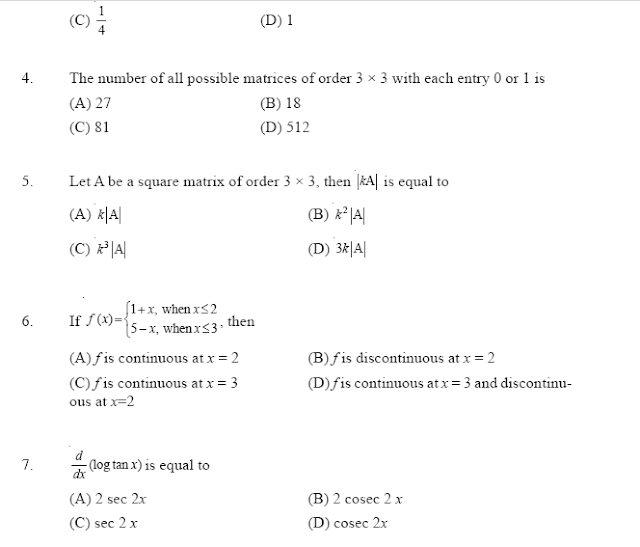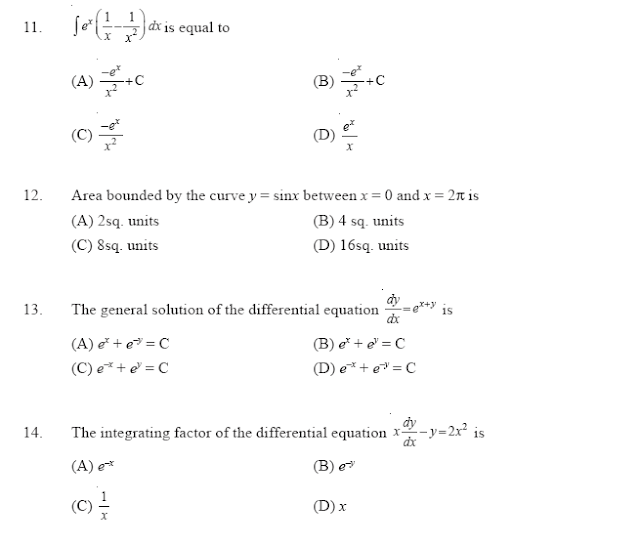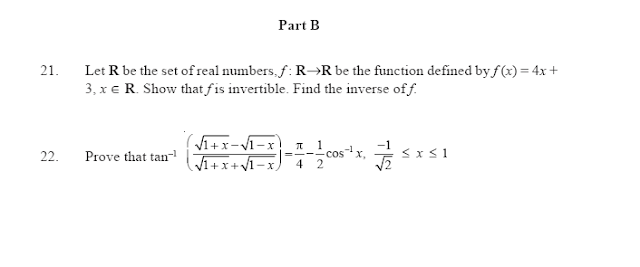# SCC Education

## CBSE Mathematics Sample paper Class 12

Time Allowed : 3 hours           Max:Marks: 100
General Instructions
(i) The question paper consists of three parts A, B and C. Each question of each
part is compulsory.
(ii) Part A Question number 1 to 20 are of 1 mark each.
(iii) Part B Question number 21 to 31 are of 4 marks each
(iv) Question number 32 to 37 are of 6 marks each
Part A
Choose the correct answer in each of the questions from 1 to 20. Each of these question contain 4 options with just one correct option.